Mathematics
Easy

Question

# The time t required to empty a water tank varies inversely as the rate of pumping p. A pump can empty a water tank in 40 min. at the rate of 120 gal/min. How long it will take the pump to empty the water tank at the rate of 200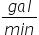?

## 48 min30 min36 min24 minHint:

## The correct answer is: 24 min

### Here, we have to find the time required to empty tha tank.We have, t =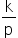40 =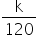…. k = 4800 t =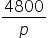=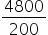= 24 min.Hence, the correct option is D.

Instead of taking y = k/x, we could also have taken xy = k, where k is a constant.

### Related Questions to study#### With Turito Foundation.#### Get an Expert Advice From Turito.